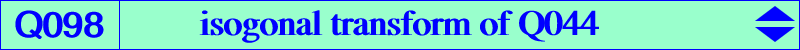too complicated to be written here. Click on the link to download a text file.X(3), X(6), X(187), X(2574), X(2575), X(3513), X(3514), X(5000), X(5001) midpoints of ABC vertices of the tangential triangle vertices of the second Brocard triangle Q1, Q2, Q3 vertices of the Thomson triangle traces of the Lemoine axis = centers of the Apollonius circles X3-OAP points, see Table 53Let A'B'C' be the circumcevian triangle of P. The lines A'B' and A'C' meet BC at Ab and Ac. The points Bc, Ba and Ca, Cb are defined likewise and these six points lie on a same conic with center Q. The points X(3), P, Q are collinear if and only if P lies on the quartic Q098. Compare with K002, property 20. See also K1065. Q098 is a circular inversible quartic with node X(3). The nodal tangents are parallel to the asymptotes of the Jerabek hyperbola. Q098 has two real asymptotes also parallel to the asymptotes of the Jerabek hyperbola. The polar line with respect to Q098 of any point lying on K626 passes through X(3). Q098 is very similar to Q023 and actually belongs to the pencil of quartics generated by Q023 and the decomposed quartic which is the union of the line at infinity and the cevian lines of X(3). Each quartic meets the circumcircle at the same points as one of the cubics of the Euler pencil. See also Q144, another analogous quartic. *** A generalization by Angel Montesdeoca It is known that the pedal triangles of two points P, Q are orthologic if and only if they are collinear with the circumcenter O of ABC. Obviously, the pedal triangles of O and P are always orthologic hence we will suppose P ≠ O in the sequel. Let then P be a fixed point and Q a variable point on the line OP with respective pedal triangles PaPbPc and QaQbQc. Denote by O1 the center of orthology of QaQbQc with respect to PaPbPc and by O2 the other center of orthology. When Q traverses the line OP, • the locus of O1 is a line (L) passing through the orthocenter Hp of PaPbPc, • the locus of O2 is a rectangular hyperbola (H) passing through P, Pa, Pb, Pc and Hp. Special cases : • (L) contains O if and only if P lies on the cubic K389. • (L) contains P if and only if P lies on Q098. • (H) contains H if and only if P lies on the cubic K376. See the general case below. Other cases : Let Po be the point on the line OP such that OPo = m/(m + n) OP (vectors). The line (L) contains Po if and only if P lies on the circular quartic Q[m,n] defined by : Q[m,n] = 16 ∆^2 m Q098 + n (x+y+z) K389 where ∆ = area(ABC). Obviously Q[m,0] = Q[1,0] = Q098 and Q[0,n] = Q[0,1] = K389 + line at infinity. In general, Q[m,n] is a circular nodal circum-quartic with node O passing through X(2574), X(2575), the vertices of the tangential triangle. If m + n ≠ 0, the nodal tangents are parallel to the asymptotes of the Jerabek hyperbola and if m + n = 0, O is a triple point on the curve Q[1,-1] with tangents parallel to the asymptotes of K006. Q[m,n] has 4 asymptotes : • two are imaginary conjugate and meet at a point X on the Euler line such that OX = (3m+n)/(6m) OX(23). This is the inverse in the circumcircle (O) of X' such that OX' = 2m/(3m+n) OH. • two are real at X(2574), X(2575) and meet at a point Y on the line through X30, X113, X1495, X1511, etc. The line XY passes through a fixed point Z, intersection of the lines 3,125 - 22,2777 - 23,1531 - 26,113 and then ZY = k ZX where the real number k is -4 cosA cosB cosC. Z is now X(22109) in ETC (2018-09-04). Q[m,n] meets (O) again at three points which are also on the Euler cubic pK(X6, S) where OS = (m+n)/(3m+n) OH. This shows that Q[1,-1] meets (O) like K003 and confirms that Q098 = Q[1,0] meets (O) like K002 since S = X(2). Note that Q[m,n] and Q023 have the same 4 points at infinity hence they must meet again at 12 other finite points that lie on a same nodal circum-cubic. For example, Q[-1,1], Q[-1,2], Q[0,1] are associated with K009, K626, K389 respectively. With Q[1,0], the cubic splits into the cevian lines of O as mentioned above. Loci related to the rectangular hyperbola (H) : (H) contains a given finite point M if and only if P lies on a cubic K(M) passing through O, M and having three real asymptotes perpendicular to the sidelines of ABC. K(M) is a circum-cubic if and only if M = H and then it is K376. K(M) meets (O) at 6 points : these are the common points (apart A, B, C) of pK(X6, M) and nK0(X6, R), where R = - a^2 SA u + SB^2 v + SC^2 w + 2 ∆^2 (u + v + w) : : . When M lies on the line GK = X(2)X(6), the cubic K(M) is a central cubic with center X on the Brocard axis OK. If GM = 2k GK then KX = k KO.